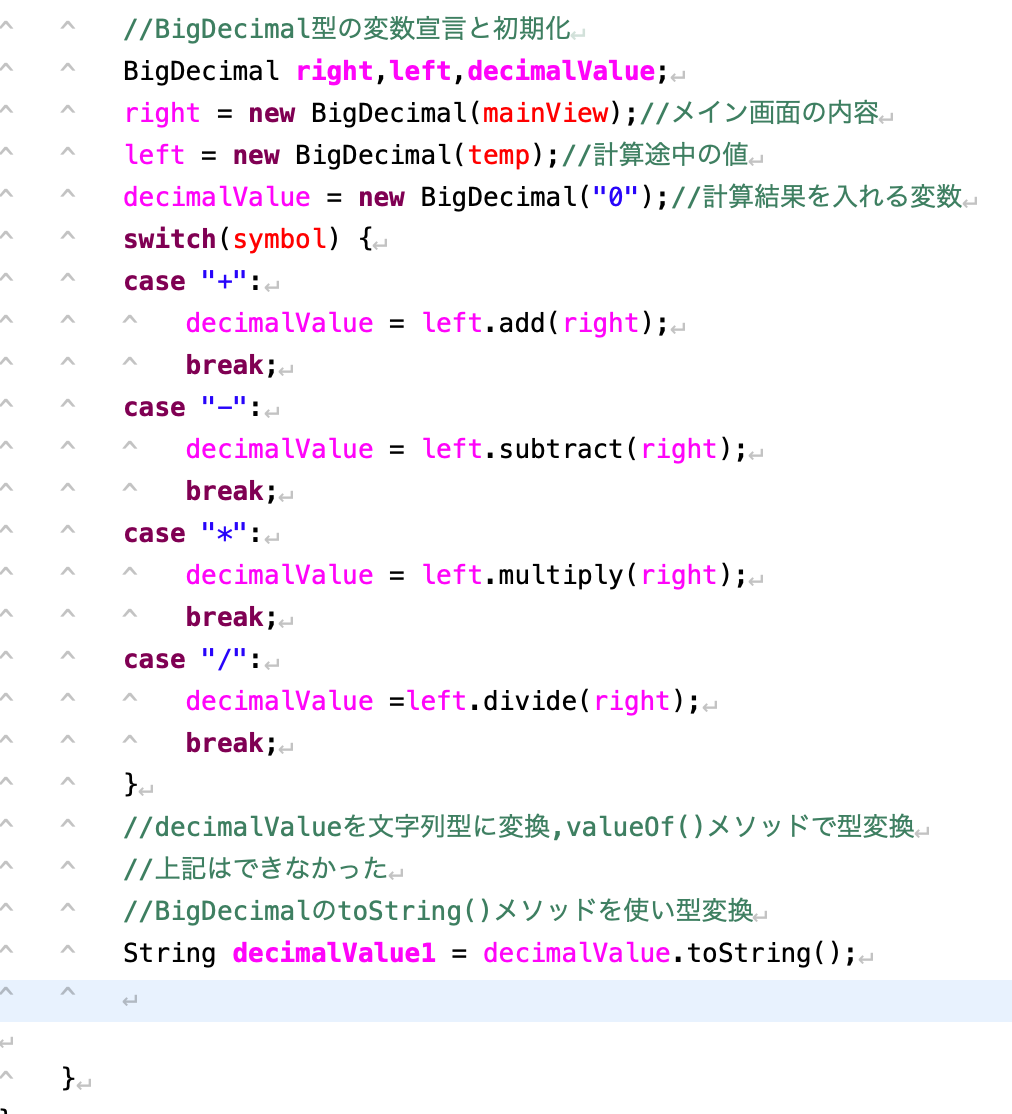# Type conversion from java BigDecimal type to String type

It seems that java originally has a class called BigDecimal.

Declaring a variable of type BigDecimal for the first time I thought that I should use the valueOf () method when I finally wanted to convert a variable of BigDecimal type to String type, but it seems that BigDecimal has a method for type conversion.

sampleFirst of all, the variable declaration & initialization of BigDecimal type is done at the top. There are 3 variables (right, left, decimalValue) in all.

Next, in the switch statement, the calculation results of right and left are stored in decimalValue.

And the point of interest is type conversion. String decimalValue1 = decimalValue.toString(); The part of is type conversion.

First, prepare decimalVlue1 to put the converted value Next, the decimalValue is converted using the method that BigDecimal has called toString ().